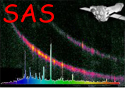XMM-Newton Science Analysis System

lcplot (lcplot-1.22.1) [xmmsas_20211130_0941-20.0.0]

# Description

lcplot produces plots of time series measured either by EPIC or by the OM. These plots show both net and background count rates. lcplot can be used to display a single time series directly or the multiple time series, produced by elcbuild for EPIC and by omlcbuild for the OM, and supplied as FITS files. Alternatively the task omthlcplot can be used to produce rate plots of the OM tracking star's intensities.

The input FITS files must contain net count rates, background count rates and the associated errors. The time series must follow a regular binning scheme (i.e. equispaced time bins) . The bin width is given by the keyword TIMEDEL.

Each light curve (net and background) of the input file is plotted on a separate page. For instance, in a PPS use, EPIC time series are given in three energy bands, so the plots will be composed of three light curve pages. On the other hand, an OM FITS file which contains only one light curve, will give a single page plot.

The task performs 1) the Chi Squared probability of constancy and/or 2) the Kolmogorov-Smirnov probability of constancy tests on the total counts. lcplot tests the null hypothesis (the source is not variable) using 1). A Chi Square fit is carried out on the count distribution and the constant distribution whose value is equal to the observed mean (both statistics and the probability are computed) using 2). A Kolmogorov-Smirnov test is carried out on the cumulative probability functions of the observed count distribution and the theoretical Gaussian distribution whose mean and variance are both equal to the observed mean count.

Results are written on a single page after the light curve(s).

Plots give time after TSTART (keyword in the FITS file) on the X-axis against count rates on the Y-axis. Please note, bins with a low exposure and therefore large errors are not plotted.

lcplot allows the user to input the device type (e.g. Xwindow, Postscript, ...) for output. The y-scale of the background counts can be forced to be the same as the source counts by setting the parameter `bkgdyscale' to `yes'. Otherwise the y-axis is scaled to the most appropriate value for the background counts.

As described in , light curves should be rebinned if necessary in order to limit the number of plotted data points to 500.

Subsections
XMM-Newton SOC -- 2021-11-30